Miscellaneous

Chapter 5 Class 12 Continuity and Differentiability
Serial order wise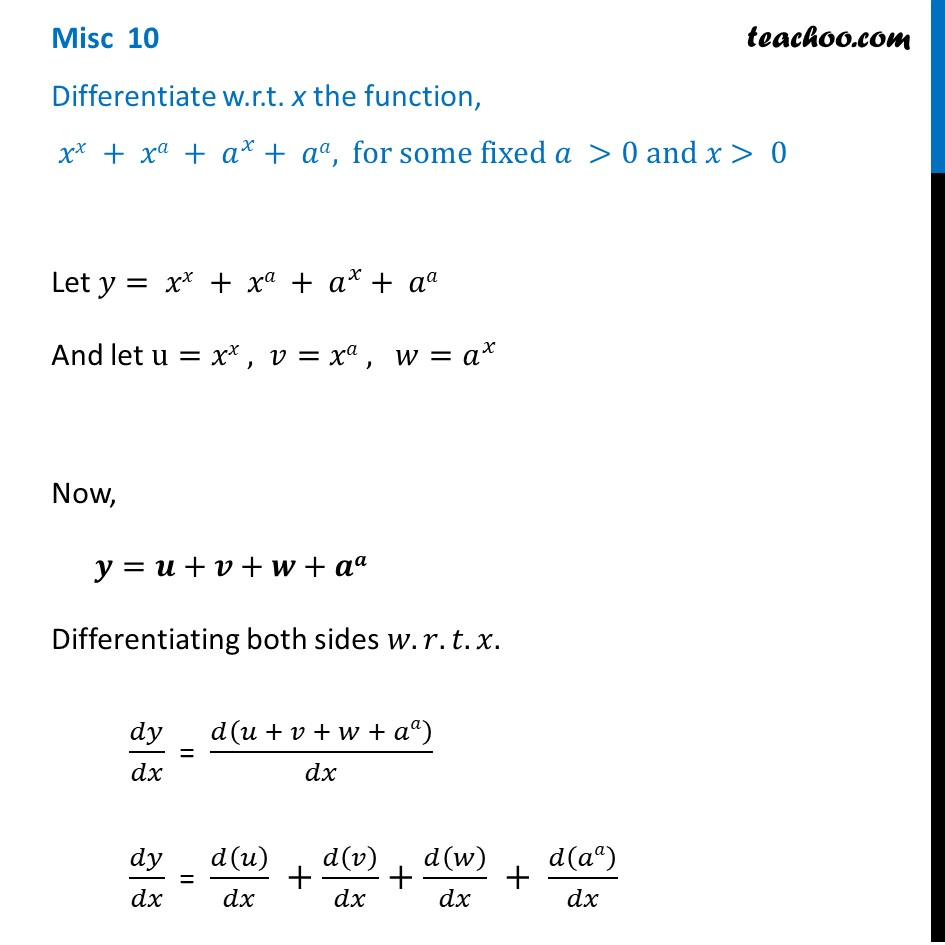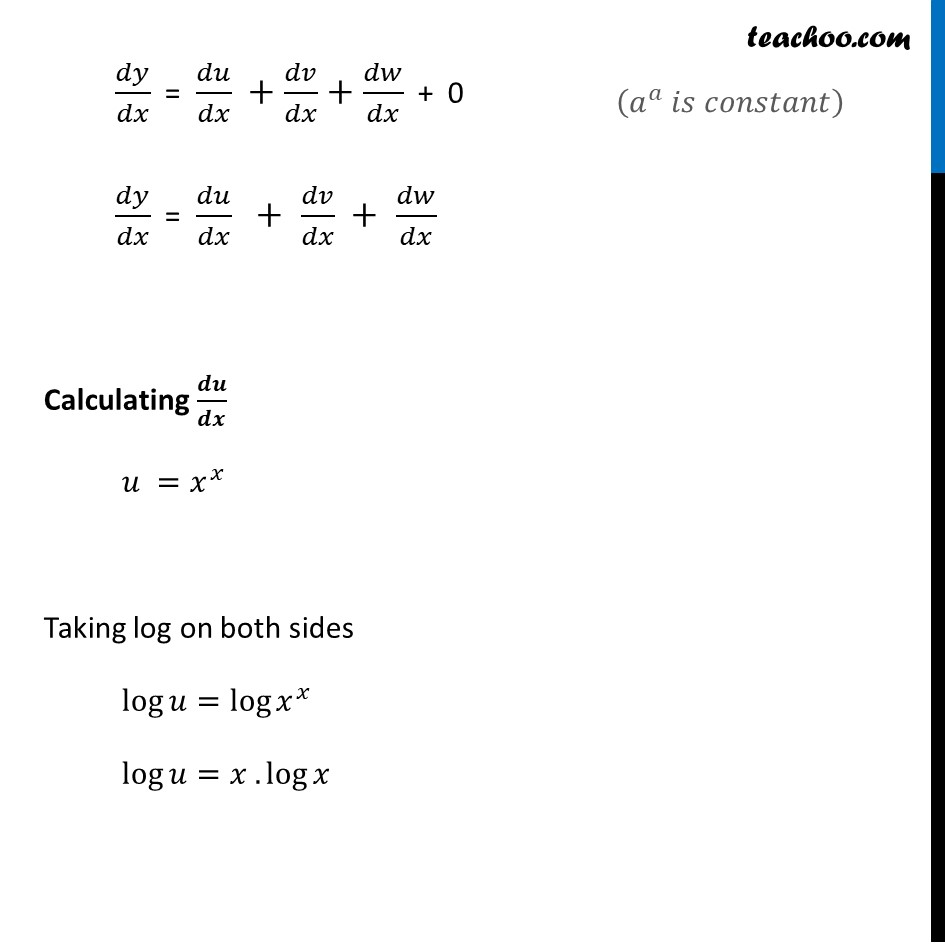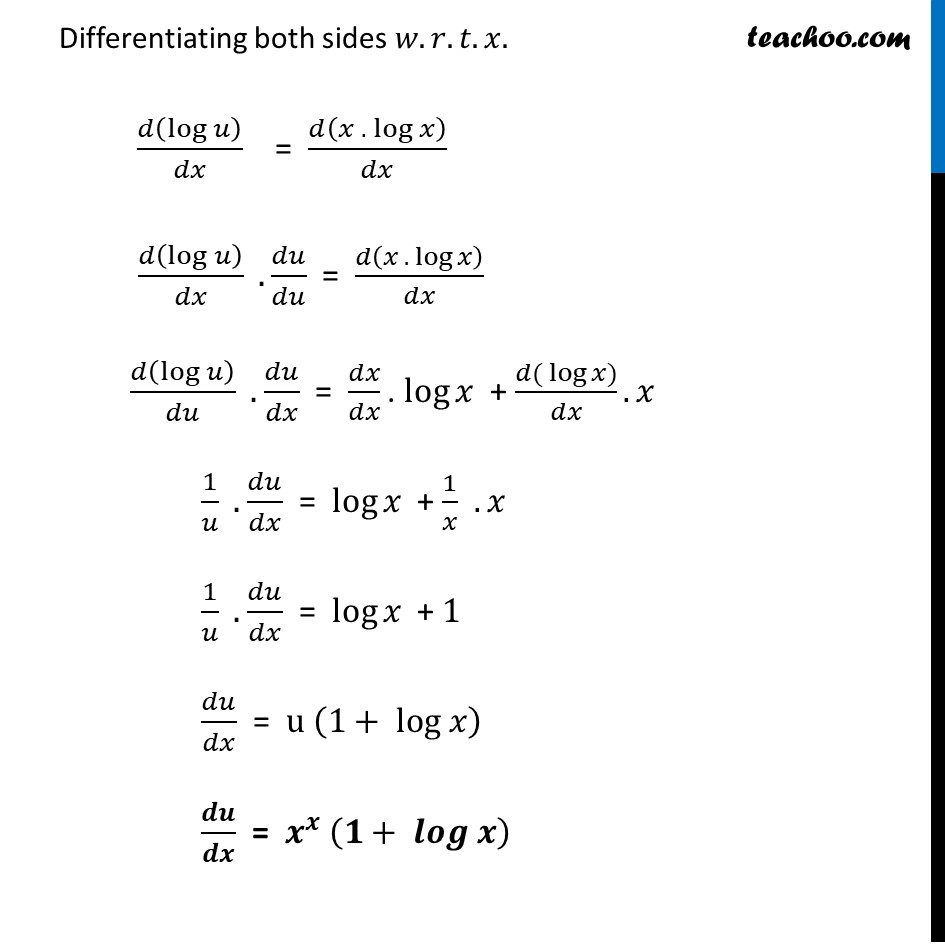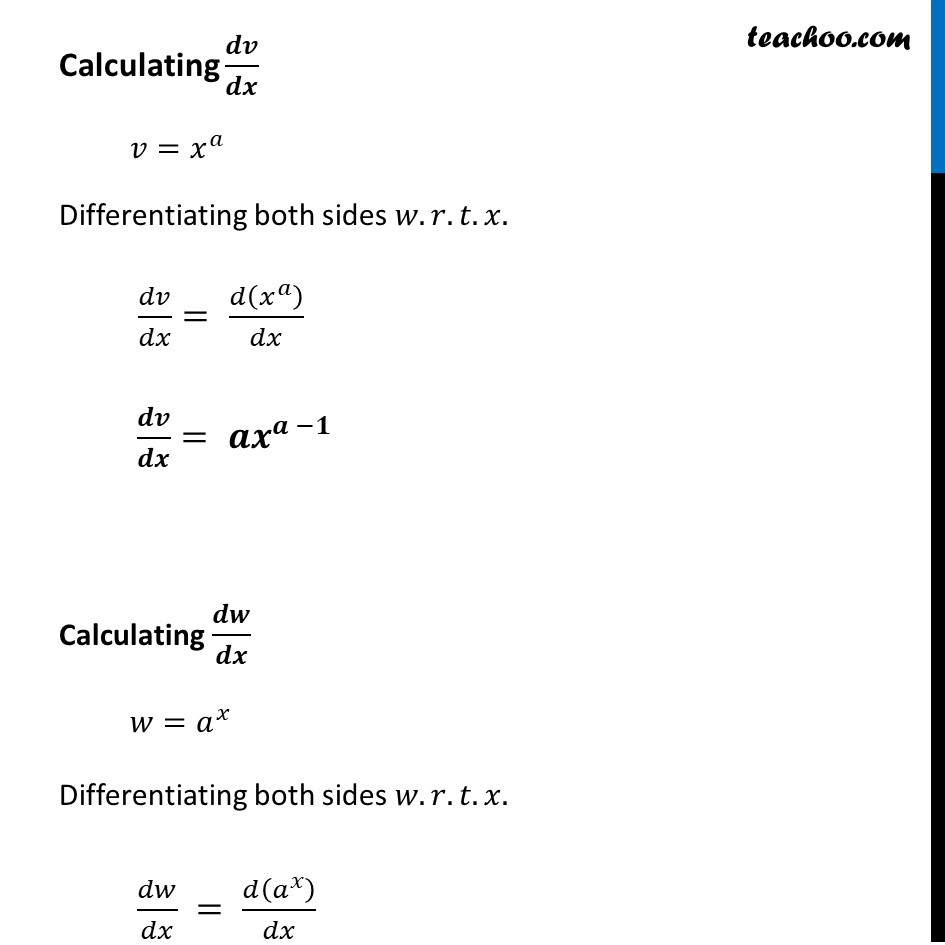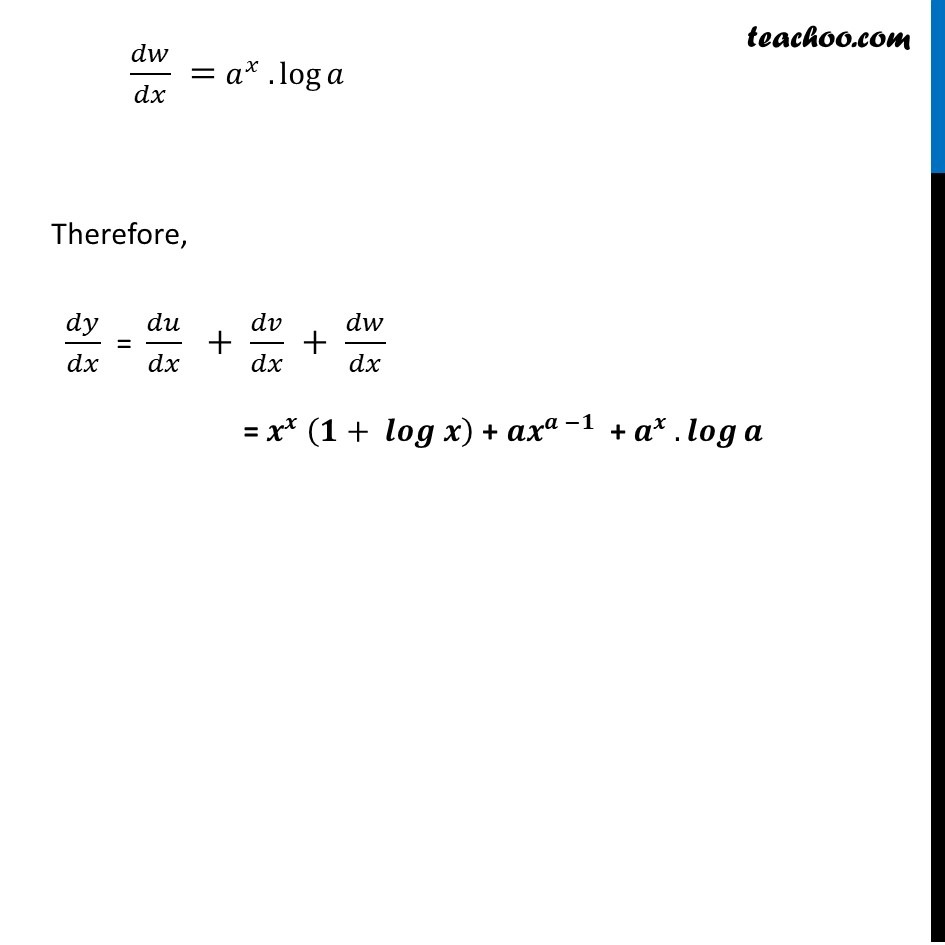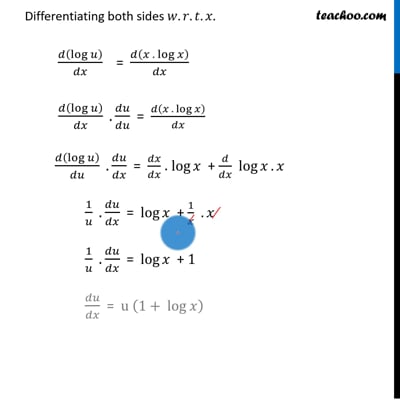This video is only available for Teachoo black users

Introducing your new favourite teacher - Teachoo Black, at only ₹83 per month

### Transcript

Misc 10 Differentiate w.r.t. x the function, 𝑥𝑥 + 𝑥𝑎 + 𝑎^𝑥+ 𝑎𝑎, for some fixed 𝑎 >0 and 𝑥> 0Let 𝑦= 𝑥𝑥 + 𝑥𝑎 + 𝑎^𝑥+ 𝑎𝑎 And let u=𝑥𝑥 , 𝑣=𝑥𝑎 , 𝑤=𝑎^𝑥 Now, 𝒚=𝒖+𝒗+𝒘+𝒂𝒂 Differentiating both sides 𝑤.𝑟.𝑡.𝑥. 𝑑𝑦/𝑑𝑥 = 𝑑(𝑢 + 𝑣 + 𝑤 + 𝑎𝑎)/𝑑𝑥 𝑑𝑦/𝑑𝑥 = 𝑑(𝑢)/𝑑𝑥 +𝑑(𝑣)/𝑑𝑥+𝑑(𝑤)/𝑑𝑥 + 𝑑(𝑎𝑎)/𝑑𝑥 𝑑𝑦/𝑑𝑥 = 𝑑𝑢/𝑑𝑥 +𝑑𝑣/𝑑𝑥+𝑑𝑤/𝑑𝑥 + 0 𝑑𝑦/𝑑𝑥 = 𝑑𝑢/𝑑𝑥 + 𝑑𝑣/𝑑𝑥 + 𝑑𝑤/𝑑𝑥 Calculating 𝒅𝒖/𝒅𝒙 𝑢 =𝑥^𝑥 Taking log on both sides log⁡𝑢=log⁡〖𝑥^𝑥 〗 log⁡𝑢=𝑥 .log⁡𝑥 (𝑎^𝑎 𝑖𝑠 𝑐𝑜𝑛𝑠𝑡𝑎𝑛𝑡) Differentiating both sides 𝑤.𝑟.𝑡.𝑥. 𝑑(log⁡𝑢 )/𝑑𝑥 = 𝑑(𝑥 .〖 log〗⁡𝑥 )/𝑑𝑥 𝑑(log⁡𝑢 )/𝑑𝑥 . 𝑑𝑢/𝑑𝑢 = 𝑑(𝑥 .〖 log〗⁡𝑥 )/𝑑𝑥 𝑑(log⁡𝑢 )/𝑑𝑢 . 𝑑𝑢/𝑑𝑥 = 𝑑𝑥/𝑑𝑥 . log⁡𝑥 + (𝑑(〖 log〗⁡𝑥))/𝑑𝑥. 𝑥 1/𝑢 . 𝑑𝑢/𝑑𝑥 = log⁡𝑥 + 1/𝑥 . 𝑥 1/𝑢 . 𝑑𝑢/𝑑𝑥 = log⁡𝑥 + 1 𝑑𝑢/𝑑𝑥 = u (1+ log 𝑥)⁡ 𝒅𝒖/𝒅𝒙 = 𝒙^𝒙 (𝟏+ 𝒍𝒐𝒈 𝒙)⁡ Calculating 𝒅𝒗/𝒅𝒙 𝑣=𝑥^𝑎 Differentiating both sides 𝑤.𝑟.𝑡.𝑥. 𝑑𝑣/𝑑𝑥= 𝑑(𝑥^𝑎 )/𝑑𝑥 𝒅𝒗/𝒅𝒙= 𝒂𝒙^(𝒂 −𝟏) Calculating 𝒅𝒘/𝒅𝒙 𝑤=𝑎^𝑥 Differentiating both sides 𝑤.𝑟.𝑡.𝑥. 𝑑𝑤/𝑑𝑥 = 𝑑(𝑎^𝑥 )/𝑑𝑥 𝑑𝑤/𝑑𝑥 = 𝑎^𝑥 .log⁡𝑎 Therefore, 𝑑𝑦/𝑑𝑥 = 𝑑𝑢/𝑑𝑥 + 𝑑𝑣/𝑑𝑥 + 𝑑𝑤/𝑑𝑥 = 𝒙^𝒙 (𝟏+ 𝒍𝒐𝒈 𝒙) + 𝒂𝒙^(𝒂 −𝟏) + 𝒂^𝒙 .𝒍𝒐𝒈⁡𝒂